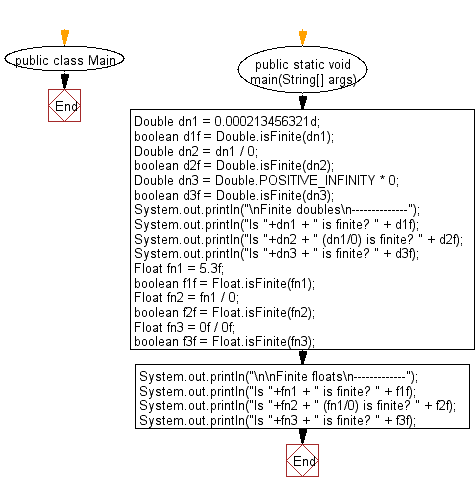﻿ Java exercises: Test whether a given double/float value is a finite floating-point value or not - w3resource# Java Data Type Exercises: Test whether a given double/float value is a finite floating-point value or not

## Java Data Type: Exercise-11 with Solution

Write a Java program to test whether a given double/float value is a finite floating-point value or not.

Sample Solution:

Java Code:

``````public class Main {
public static void main(String[] args) {
Double dn1 = 0.000213456321d;
boolean d1f = Double.isFinite(dn1);
Double dn2 = dn1 / 0;
boolean d2f = Double.isFinite(dn2);
Double dn3 = Double.POSITIVE_INFINITY * 0;
boolean d3f = Double.isFinite(dn3);
System.out.println("\nFinite doubles\n--------------");
System.out.println("Is "+dn1 + " is finite? " + d1f);
System.out.println("Is "+dn2 + " (dn1/0) is finite? " + d2f);
System.out.println("Is "+dn3 + " is finite? " + d3f);
Float fn1 = 5.3f;
boolean f1f = Float.isFinite(fn1);
Float fn2 = fn1 / 0;
boolean f2f = Float.isFinite(fn2);
Float fn3 = 0f / 0f;
boolean f3f = Float.isFinite(fn3);
System.out.println("\n\nFinite floats\n-------------");
System.out.println("Is "+fn1 + " is finite? " + f1f);
System.out.println("Is "+fn2 + " (fn1/0) is finite? " + f2f);
System.out.println("Is "+fn3 + " is finite? " + f3f);
}
}
```
```

Sample Output:

```inite doubles
--------------
Is 2.13456321E-4 is finite? true
Is Infinity (dn1/0) is finite? false
Is NaN is finite? false

Finite floats
-------------
Is 5.3 is finite? true
Is Infinity (fn1/0) is finite? false
Is NaN is finite? false
```

Flowchart:Java Code Editor:

Improve this sample solution and post your code through Disqus

What is the difficulty level of this exercise?

﻿

## Java: Tips of the Day

Checks if a string is upper case:

```public static boolean isUpperCase(String input) {
return Objects.equals(input, input.toUpperCase());
}
```

Ref: https://bit.ly/39Hpo84